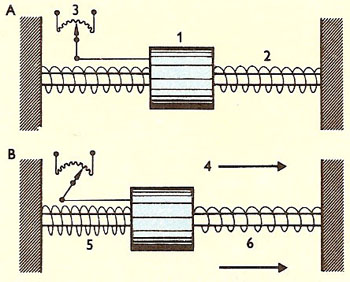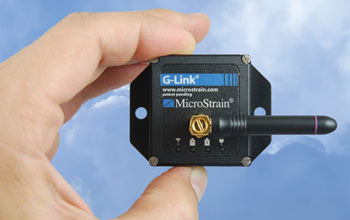A# accelerationA linear accelerometer. If the device is moving at constant velocity, the mass (1), supported on a bar by springs (2), remains static and an intermediate reading is registered on the potentiometer (3). On acceleration in the direction of the bar (4), i.e., along the accelerometer's sensitive axis, inertia causes the mass to lag behind, compressing the spring behind it (5) and stretching the spring ahead of it (6): a high voltage is registered. On deceleration, inertia causes the mass to compress the spring ahead of it and stretch that behind it, and thus a low voltage is registered on the potentiometer.Developed by Steve Arms and his colleagues at MicroStrain, the G-LINK Wireless Accelerometer nodes monitored vibration and shock loads within the Liberty Bell during its relocation. Image credit: MiocroStrain, Inc. Caption text: NSF.

Acceleration is the rate at which the velocity of an object changes with time. Acceleration is a vector quantity and may involve a change in an object's speed or direction or both. Deceleration is considered to be negative acceleration.

For a body moving in a straight line with constant acceleration a, from initial speed u to final speed v, the acceleration is given by

a = (v - u)/t = (v 2 - u 2)/2s

where t is the time taken and s is the distance covered.

If the acceleration is not constant it is given by dv/dt = d 2s/dt 2.

Linear acceleration occurs when the rate of motion (speed) along a straight line changes. Centripetal acceleration occurs in circular motion at constant angular velocity as the direction of motion changes. Angular acceleration occurs in circular motion when the rate of rotation changes.

Acceleration is the result of a (net) force (F) applied to an object (of mass m). We can therefore express acceleration in terms of an applied force through this equation derived from Newton's second law: a = F/m.

The SI unit for acceleration is meter per second squared (m/s2).

## Angular acceleration

Angular acceleration is the rate of change of angular velocity. The average angular acceleration of an object whose angular velocity changes from ω1 to ω2 over time t is (ω2 - ω1)/t. Instantaneous acceleration is the value approached as t becomes small. The direction of the angular acceleration vector is perpendicular to the plane of motion. The tangential acceleration aT of a particle at a distance r from a fixed point is , where α is its angular acceleration.

## Accelerometer

An accelerometer is an instrument that measures acceleration or the gravitational force capable of imparting acceleration. It usually employs a concentrated mass that resists movement because of its inertia; acceleration is measured in terms of the displacement of this mass relative to its supporting frame or container.

The simplest example of an accelerometer is a plumb bob suspended from the object being accelerated. The angle of the plumb bob is proportional to the acceleration. A more sophisticated version, used in ballistic missiles, is an electromechanical device that translates the acceleration into electric current.

Modern accelerometers are typically micro-machined silicon sensors that are based on the capacitive, piezo-resistive or optical detection of the deflection a small mass experiences when the sensors is subjected to acceleration.